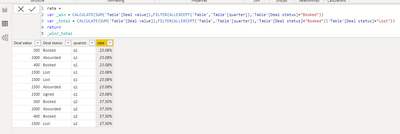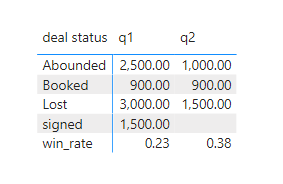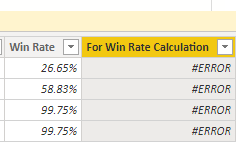cancel
Showing results for
Did you mean:Helper I

## Win Rate

Hi there!

In my data query I have a table with the 'Sales Information', includes the following columns:

 Deal value Deal status 500 Booked 1000 Abounded 400 Booked 1500 Lost 2000 Lost 1500 Abounded 4000 signed

In the report view, I already created a table that includes the sum of the values for ‘Deal Status’ by every quarter…

but I still want to add the win rate to the table for every quarter exactly as follow:

 Q1 Q2 Q3 Q4 Booked 10 12 11 14 Signed 1 2 3 4 Lost 14 15 16 18 Abounded 1 2 3 4 Win Rate 42% 44% 41% 44%

Win rate calculation = sum of [deal value] for the booked [deal status]/sum of [deal value] for the booked [deal status]+sum of [deal value] for the Lost [deal status].

What do you think the best way to apply this?

I hope it is easy for you to help.

1 ACCEPTED SOLUTIONCommunity Support

Create a calculated column like below.

``````rate =
var _win = CALCULATE(SUM('Table'[Deal value]),FILTER(ALLEXCEPT('Table','Table'[quarter]),'Table'[Deal status]="Booked"))
var _total = CALCULATE(SUM('Table'[Deal value]),FILTER(ALLEXCEPT('Table','Table'[quarter]),'Table'[Deal status]="Booked"||'Table'[Deal status]="Lost"))
return
_win/_total``````Then create a new table:

``````Table 2 =
var _table1 = SELECTCOLUMNS('Table',"deal status",'Table'[Deal status],"deal value",'Table'[Deal value],"quarter",[quarter])
var _table2 = distinct(SELECTCOLUMNS('Table',"deal status","win_rate","deal value",'Table'[rate],"quarter",[quarter]))
return
UNION(_table1,_table2)``````Then use this table to create matrix.Best Regards,

Jay

Community Support Team _ Jay
If this post helps, then please consider Accept it as the solution
to help the other members find it.
8 REPLIES 8Helper I

Hi @v-jayw-msft ,

i wolud like to thank you for answering my query perfictly,

But I need some additional help over here.

I added an additional colomn to the final table, just to link it with the fact table.

But i am getting the following error:

A circular dependency was detected.

have you ever experinced this kind of issues?

do you have any idea how can i solve it?Community Support

Create a calculated column like below.

``````rate =
var _win = CALCULATE(SUM('Table'[Deal value]),FILTER(ALLEXCEPT('Table','Table'[quarter]),'Table'[Deal status]="Booked"))
var _total = CALCULATE(SUM('Table'[Deal value]),FILTER(ALLEXCEPT('Table','Table'[quarter]),'Table'[Deal status]="Booked"||'Table'[Deal status]="Lost"))
return
_win/_total``````Then create a new table:

``````Table 2 =
var _table1 = SELECTCOLUMNS('Table',"deal status",'Table'[Deal status],"deal value",'Table'[Deal value],"quarter",[quarter])
var _table2 = distinct(SELECTCOLUMNS('Table',"deal status","win_rate","deal value",'Table'[rate],"quarter",[quarter]))
return
UNION(_table1,_table2)``````Then use this table to create matrix.Best Regards,

Jay

Community Support Team _ Jay
If this post helps, then please consider Accept it as the solution
to help the other members find it.Helper I

Hi @v-jayw-msft ,

The first table created perfectly,  but when I come to the second table, it brings Error data with the folloing message:Please note that I copied the same function as yours.Helper I

Hi @v-jayw-msft !

Did you get any solutin for my problem?

Thanks a lot!Community Support

Please split the second table into two tables.
table1 = SELECTCOLUMNS(...
table2 = distinct(...
Then check which table occurred the error.

It looks like there are some differences between your raw data and the sample data.

I didn't get this error when using the data you provided above so that I could not reproduce the scenario.

Best Regards,

Jay

Community Support Team _ Jay
If this post helps, then please consider Accept it as the solution
to help the other members find it.Super User

@Hadill , You need have measure for booked , lost , others and win %

Win Ratio =

Divide( Calculate(Sum(Table[Deal Amount] ), Filter(Table, Table[Status] ="Booked")) ,Calculate(Sum(Table[Deal Amount] ), Filter(Table, Table[Status] in{"Booked","Lost"} )))

You can use Show on row in  matrix visual

Or you can show win % in Grand Total

If (isinscope(Table[Status]) ,Sum(Table[Deal Amount] ) , [Win Ratio])

https://www.kasperonbi.com/use-isinscope-to-get-the-right-hierarchy-level-in-dax/Helper I

I think the second option cannot be applied in my matrix case.

the first solution is applicable, but unfortunately it brings the grand total value for all the years and quarters.

I need to show the data with quarter regards.

I hope you can help in thisSuper User

@Hadill , In the first option you have all the measures and those should follow the filter on columns and in slicer.

You will have total on column

In second case you will replace the total columnAnnouncements#### Exclusive opportunity for Women!

Join us for a free, hands-on Microsoft workshop led by women trainers for women where you will learn how to build a Dashboard in a Day!#### Power Platform Conference-Power BI and Fabric Sessions

Join us Oct 1 - 6 in Las Vegas for the Microsoft Power Platform Conference.Top Solution Authors
Top Kudoed Authors
Users online (2,457)